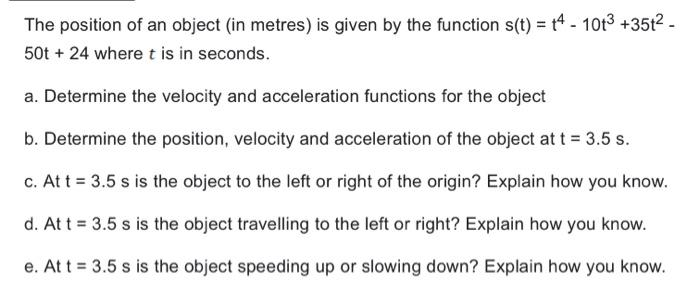Home / Expert Answers / Calculus / the-position-of-an-object-in-metres-is-given-by-the-function-s-t-t-4-10-t-3-35-t-2-pa591

# (Solved): The position of an object (in metres) is given by the function $$s(t)=t^{4}-10 t^{3}+35 t^{2}-$$ ...The position of an object (in metres) is given by the function $$s(t)=t^{4}-10 t^{3}+35 t^{2}-$$ $$50 \mathrm{t}+24$$ where $$t$$ is in seconds. a. Determine the velocity and acceleration functions for the object b. Determine the position, velocity and acceleration of the object at $$t=3.5 \mathrm{~s}$$. c. At $$t=3.5 \mathrm{~s}$$ is the object to the left or right of the origin? Explain how you know. d. At $$t=3.5 \mathrm{~s}$$ is the object travelling to the left or right? Explain how you know. e. At $$t=3.5 \mathrm{~s}$$ is the object speeding up or slowing down? Explain how you know.

We have an Answer from Expert